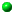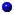\item Prove that the quadratic form $q(x) = x_1^2 + x_2^2 - p (x_3^2 + x_4^2)$ does not represent zero, when $p=3$ or more generally when $p$ is a prime congruent to $3 \mod 4$.

### Topics in Geometry and DynamicsMath 275 - Harvard UniversitySyllabus and referencesNotes on 3-manifolds!Course notesProblemsDiscussion Group: Fridays, 12:30, 4th Floor CommonsHomework EssaysHistory of the ergodic theoremFrequency of different decimal digits